# Dimensions welcomes award-winning photo-based artist Lora Moore-Kakaletris

For Lora Moore-Kakaletris, photography is so much more than understanding the intricacies of the latest camera gear. "It is my tool for expressing my deepest fears, my greatest joys, my deepest thoughts. It is my instrument for dealing with and expressing my feelings of loss, grief, joy and love.

"Photography makes me stay up late at night and jump out of bed early in the morning. I am overwhelmed with the drive - the need - to share my perspective of the world around me and to leave a permanent mark: a body of work that says I was here and this is my story."Couch Potato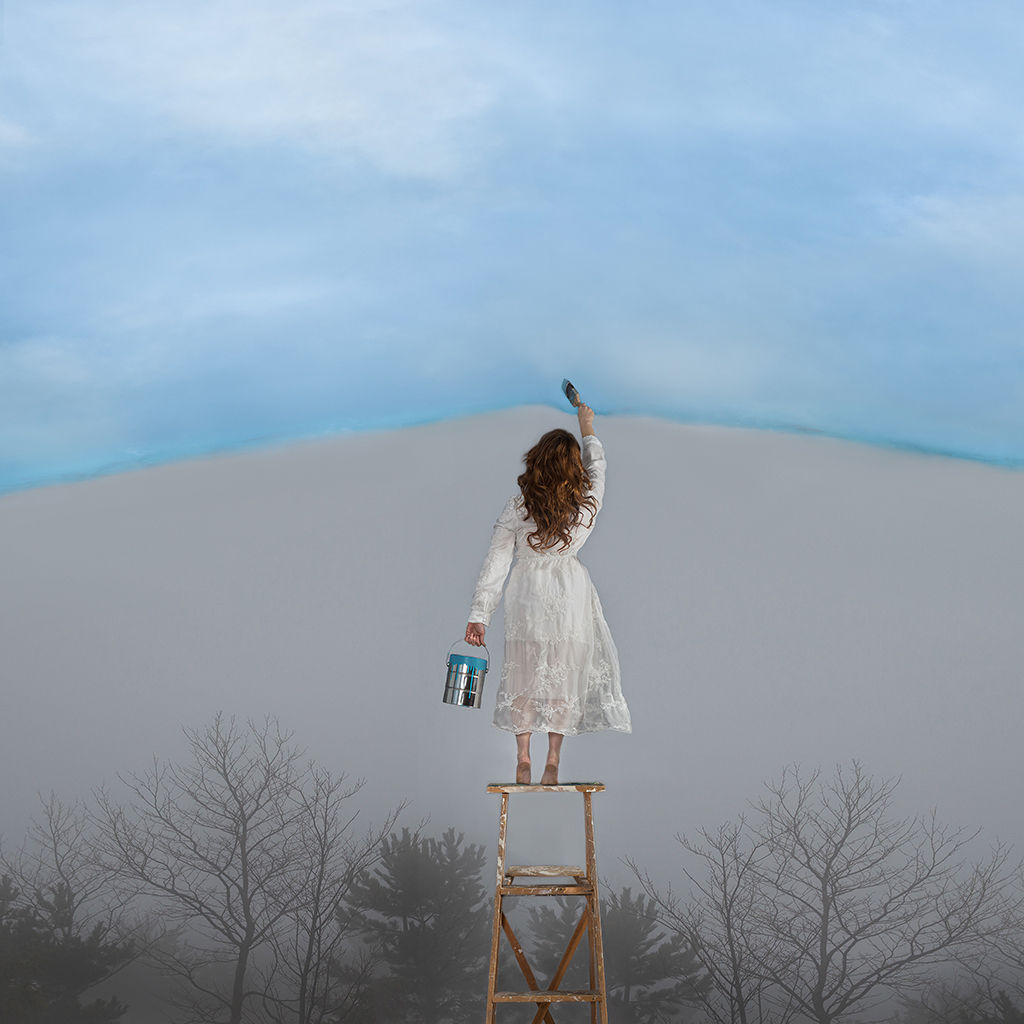Blue Skies Ahead
 table div table+table+table+table+table+table div table{width:100%;padding:0}table div table+table+table+table+table+table div table img{width:96.23%;padding:0;float:none}table div table+table+table+table+table+table div table td{width:100%;padding:0 1.88% 18px}/* styles */# NEW works by Peter Andrew Lusztyk

"I want you to see the world as I see it," says photographer Peter Andrew Lusztyk. "I want you to be amazed." For Peter, "photography is the process of turning ordinary or overlooked things into things that cause you to pause and examine; things that make your eyes explode because they are so incredibly beautiful."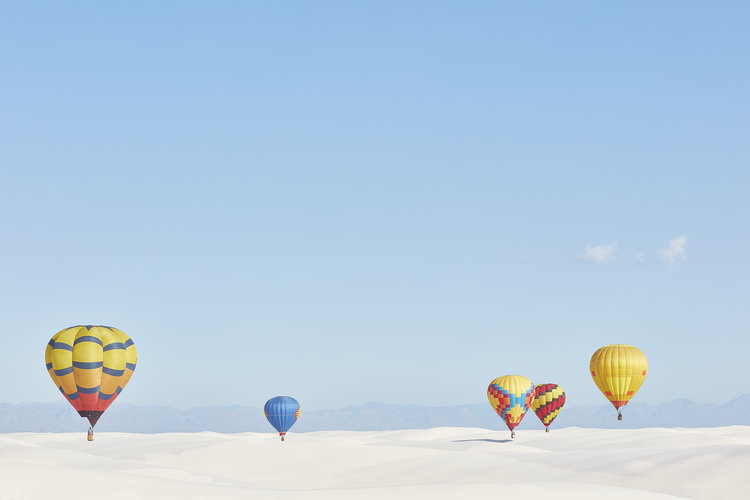Balloons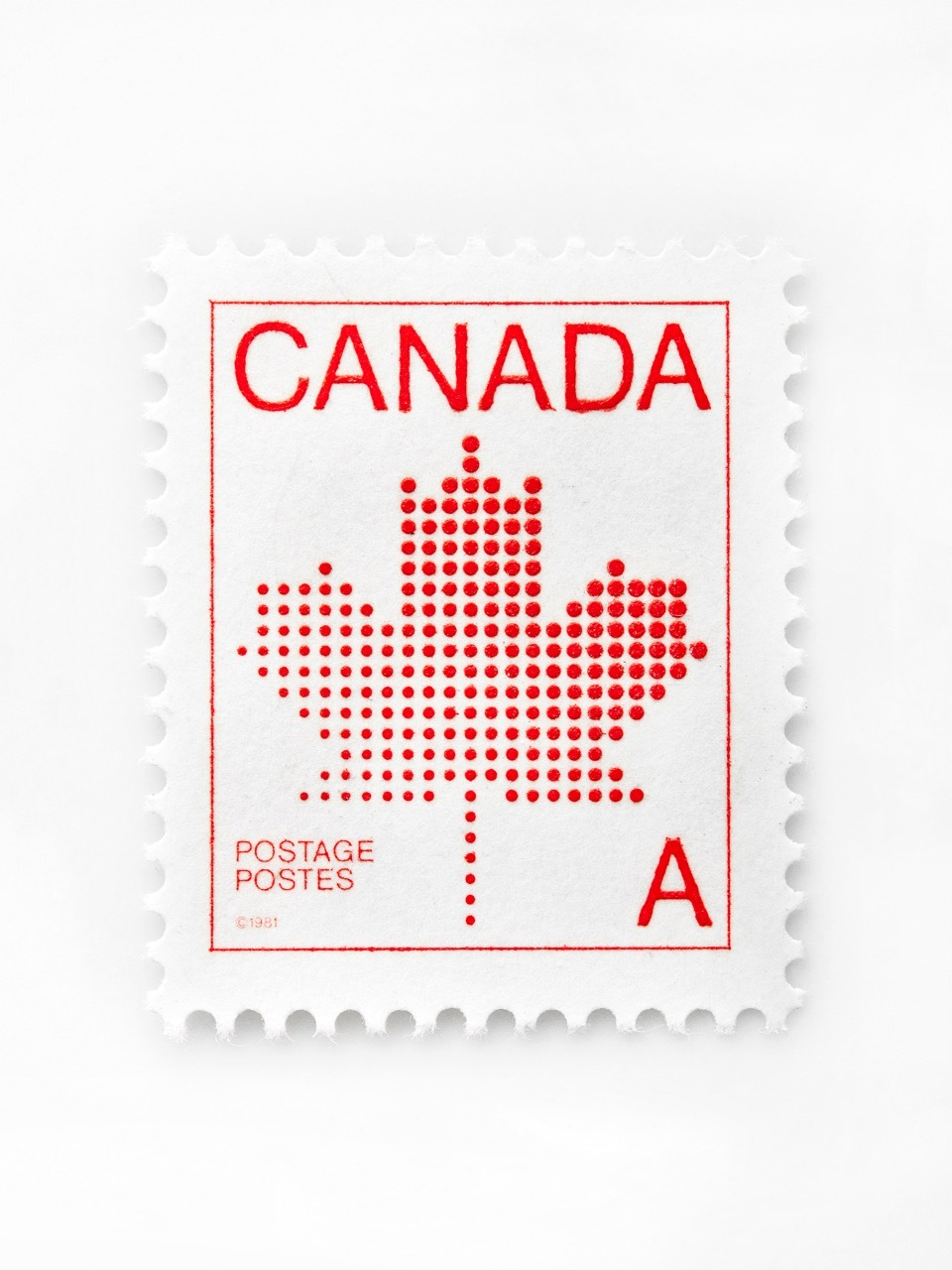1981 30c stamp
 table div table+table+table+table+table+table+table+table+table+table div table{width:100%;padding:0}table div table+table+table+table+table+table+table+table+table+table div table img{width:96.23%;padding:0;float:none}table div table+table+table+table+table+table+table+table+table+table div table td{width:100%;padding:0 1.88% 18px}/* styles */# Cody Greco Matchbox series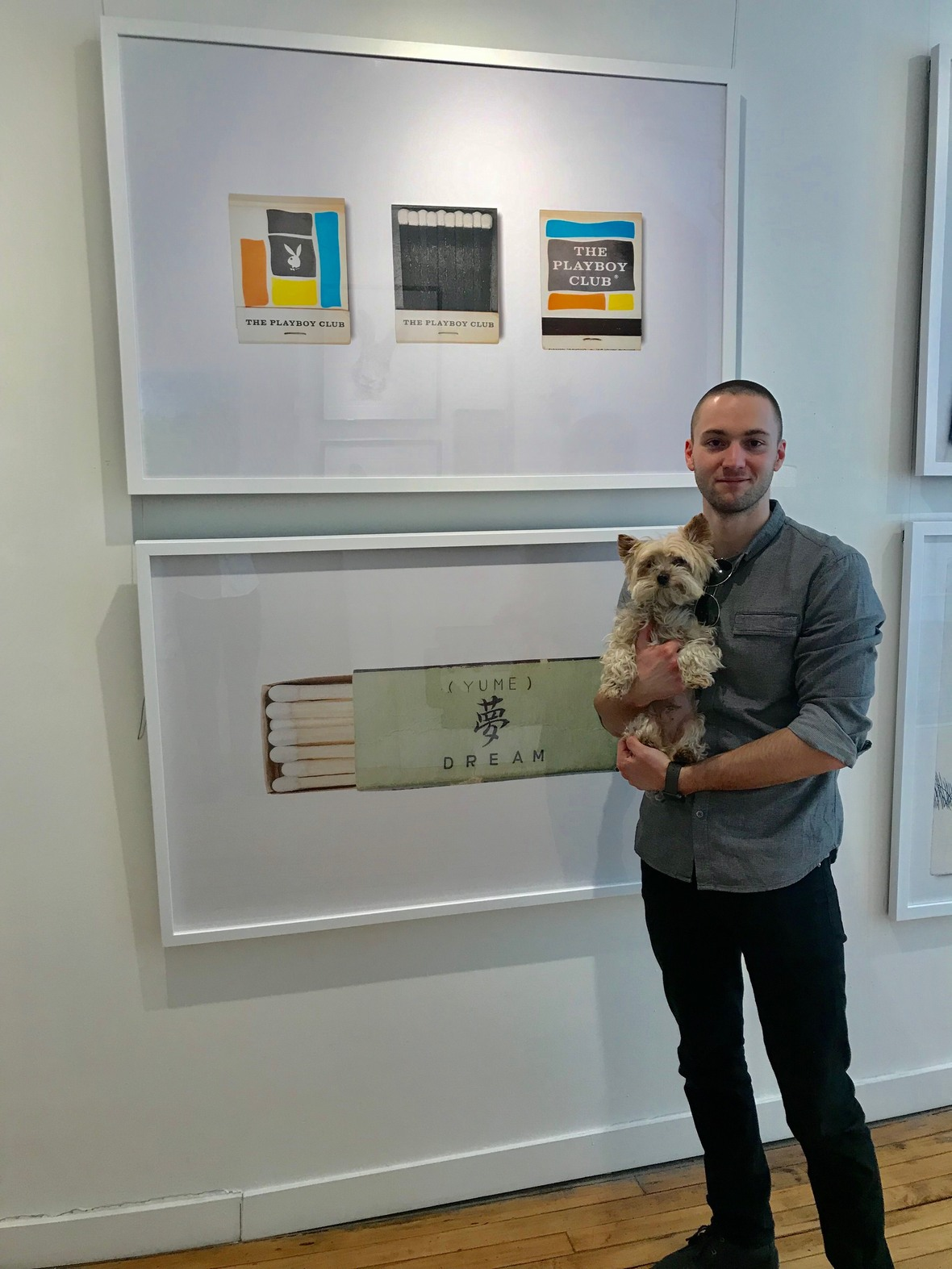Cody Greco with Hudson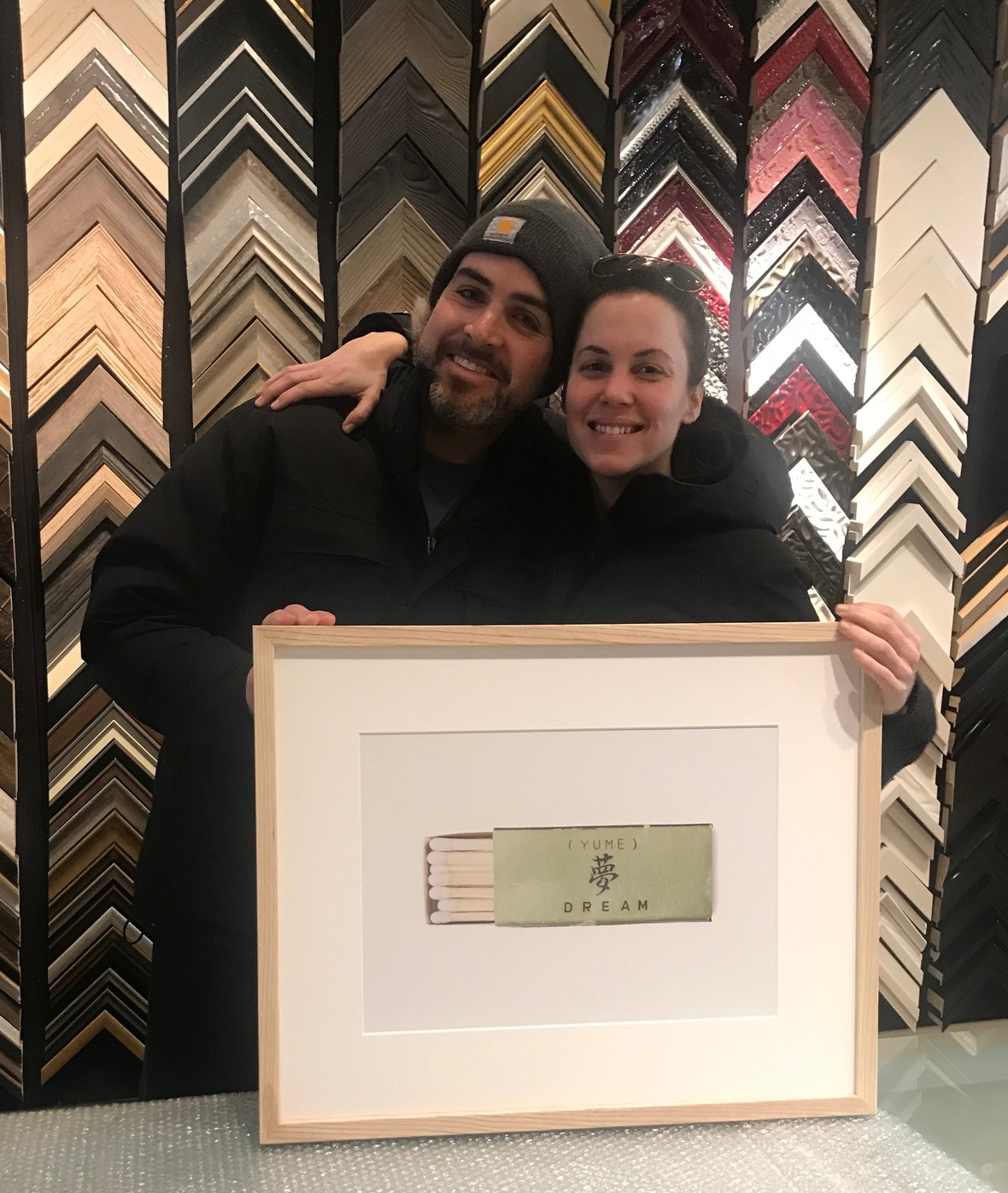Happy clients!
 table div table+table+table+table+table+table+table+table+table+table+table+table+table div table{width:100%;padding:0}table div table+table+table+table+table+table+table+table+table+table+table+table+table div table img{width:96.23%;padding:0;float:none}table div table+table+table+table+table+table+table+table+table+table+table+table+table div table td{width:100%;padding:0 1.88% 18px}/* styles */# Mom's special day is Sunday, May 12

Say "I love you" with something special this Mother's Day - a one-size-fits-all gift certificate from Dimensions guaranteed to please the mom(s) in your life!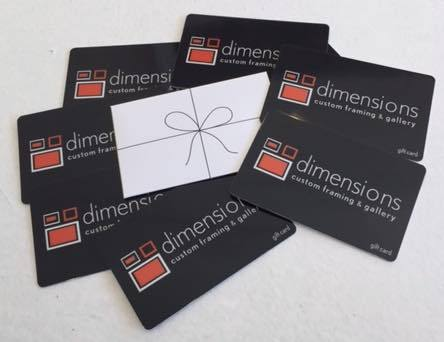table div table+table+table+table+table+table+table+table+table+table+table+table+table+table+table+table div table{width:100%;padding:0}table div table+table+table+table+table+table+table+table+table+table+table+table+table+table+table+table div table img{width:96.23%;padding:0;float:none}table div table+table+table+table+table+table+table+table+table+table+table+table+table+table+table+table div table td{width:100%;padding:0 1.88% 18px}/* styles */# Wendy, Ellen, Hudson

Visit us online @ dimensionsframing.com

# Stay connected with us!

 table div table+table+table+table+table+table+table+table+table+table+table+table+table+table+table+table+table+table div table{width:100%;padding:0}table div table+table+table+table+table+table+table+table+table+table+table+table+table+table+table+table+table+table div table img{width:96.23%;padding:0;float:none}table div table+table+table+table+table+table+table+table+table+table+table+table+table+table+table+table+table+table div table td{width:100%;padding:0 1.88% 18px}/* styles */table div table+table+table+table+table+table+table+table+table+table+table+table+table+table+table+table+table+table+table+table+table div table{width:100%;padding:0}table div table+table+table+table+table+table+table+table+table+table+table+table+table+table+table+table+table+table+table+table+table div table img{width:96.23%;padding:0;float:none}table div table+table+table+table+table+table+table+table+table+table+table+table+table+table+table+table+table+table+table+table+table div table td{width:100%;padding:0 1.88% 18px}/* styles */